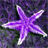# Draw a polygon programatically in ArcGlobe

591
0
10-10-2013 07:42 AMNew Contributor
All,

I need some very basic guidance.  I am trying to draw a polygon on the globe in ArcGlobe programatically.  I have the four corner lat/lon coordaintes of the polygon.  I have looked at the snippet Add Graphics Layer to Globe and I believe I saw some sample code to do this using OpenGL but would prefer to do something similar to the Add Graphics Layer to Globe snippet.  Here's the code I have so far.  Please let me know if I am way off or not.  Thanks!

```        //Create a new polygon
IElement polygonElement = new PolygonElementClass();
//Set the geometry
ESRI.ArcGIS.Geometry.IPoint ul = new ESRI.ArcGIS.Geometry.PointClass();
ul.X = -77.198348;
ul.Y = 38.746170;
ESRI.ArcGIS.Geometry.IPoint ur = new ESRI.ArcGIS.Geometry.PointClass();
ur.X = -77.195175;
ur.Y = 38.748786;
ESRI.ArcGIS.Geometry.IPoint lr = new ESRI.ArcGIS.Geometry.PointClass();
lr.X = -77.192939;
lr.Y = 38.746437;
ESRI.ArcGIS.Geometry.IPoint ll = new ESRI.ArcGIS.Geometry.PointClass();
ll.X = -77.195343;
ll.Y = 38.744491;
ESRI.ArcGIS.Geometry.IPointCollection ring =
new ESRI.ArcGIS.Geometry.RingClass();
ring.AddPoint(ul);
ring.AddPoint(ur);
ring.AddPoint(lr);
ring.AddPoint(ll);
//Add the geometry
ESRI.ArcGIS.Geometry.IGeometryCollection polygon =
new ESRI.ArcGIS.Geometry.PolygonClass();
polygon.AddGeometry(ring as ESRI.ArcGIS.Geometry.IGeometry);
// Add to the graphics layer
polygonElement.Geometry = ((ESRI.ArcGIS.Geometry.IGeometry)polygon);
((IGraphicsContainer)m_globeGraphicsLayer).AddElement(polygonElement, 1);```
Tags (5)
0 Replies